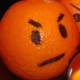# Leet More 2010 Time Traveller writeup

A scientist who worked on the issue of space-time continuum, suddenly disappeared.
He left only
a mysterious drawing in a notebook.
There is a suspicion that he traveled back in time. In what year did he travel? And what is his name?There are only 2 breaks in this maze. Let’s try to find the way. Ascii of lines length don’t mean anything, but turns do. Each turn is a bit. Just experiement to find that turn right = 1, turn left = 0. Here is a script to collect bits:

<?php

$im = imagecreatefrompng($argv);
if (!$im) die("can't open image\n");$right1 = 1; #if turn right = 1
$sx =$sy = 512;

#start coordinates
$x = 256;$y = 246;
$angle = 270;$key = '';
while (1) {
$cos = intval(cos(deg2rad($angle)));
$sin = intval(sin(deg2rad($angle)));
$c = imagecolorat($im, $x+$cos, $y-$sin);
if ($c) #clear pixels for doctor's name imagesetpixel($im, $x+$cos, $y-$sin, 0x30);
if (!$c) {$ar = ($angle + 270 ) % 360;$al = ($angle + 90 ) % 360;$xr = $x+intval(cos(deg2rad($ar)));
$yr =$y-intval(sin(deg2rad($ar)));$xl = $x+intval(cos(deg2rad($al)));
$yl =$y-intval(sin(deg2rad($al)));$cr = imagecolorat($im,$xr, $yr);$cl = imagecolorat($im,$xl, $yl); if ($cl && $cr) die("Picture failish\n"); if (!$cl && !$cr) break; if ($cr) {
$angle =$ar;
$x =$xr;
$y =$yr;
}
else {
$angle =$al;
$x =$xl;
$y =$yl;
}
$key .= (!!($cr) ^ $right1) ? 1 : 0; } else {$x += $cos;$y -= $sin; //axes differ } }$a = str_split($key, 8); foreach ($a as $b) { echo chr(bindec($b));
}
echo "\n";

imagepng($im, "out.png"); ?> $ php solve.php traveller.png
Th1RtY_e1gHT_5ev3nTY_0Ne_A.D.

out.png contains a shadowed path and we can see the scientist’s name:So, the answer to Time Traveller 2 was “Dr Leet”

•• unkn0w on June 15, 2011 at 11:07

interesting challenge, but the question is how we can build such as image ?
is there some trick using grafis editor or it just with the code ?
if you please let me know ?

•• hellman on June 15, 2011 at 13:07
Author

I built it with a script on php. It generates 100 random pics with the hidden text. So you can choose a nice one.

“Dr Leet” text was added later manually.

•• unkn0w on June 15, 2011 at 23:44

how we add the Dr Leet text ?
first we add the text to an image or text added after we generated an image ?

•• hellman on June 16, 2011 at 11:12
Author

First we generate the image, then we add Dr Leet text

•• unkn0w on June 16, 2011 at 11:28

thanks sir, i have try it and it so awesome, but a little hard to add the text on that image so that people cant notice there is secret text inside an image, i use photoshop to add the text, but mine not look so awesome as your sir, may be i should to find some font type or draw it by my self,

thanks again sir :)

•• hellman on June 16, 2011 at 11:34
Author

Yes, we drew it manually, line by line in paint :)